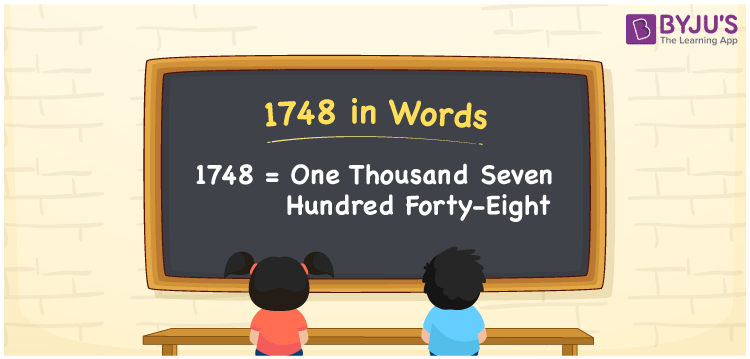# 1748 in Words

In English words, the number 1748 is represented as one thousand seven hundred forty-eight. For instance, if you have purchased the notebooks for Rs. 1748, it can be written as “I have purchased the notebooks for Rs. One thousand seven hundred forty-eight”. Also, the number 1748 is a natural number. Now, let us have a look at the procedure of writing the number 1748 in words using the place value system.

 1748 in Words: One Thousand Seven Hundred Forty-eight. One Thousand Seven Hundred Forty-eight in Numerical Form: 1748.

## 1748 in English Words## How to Write 1748 in Words?

Learn the spelling of 1748 in words using the place value table, which is provided below.

 Thousands Hundreds Tens Ones 1 7 4 8

The expanded form of 1748 is as follows:

= 1 × Thousand + 7 × Hundred + 4 × Ten + 8 × One

= 1 × 1000 + 7 × 100 + 4 × 10 + 8 × 1

= 1000 + 700 + 40 + 8

= 1748

= One thousand seven hundred forty-eight

Hence, 1748 in words is one thousand seven hundred forty-eight.

1748 in words – One thousand seven hundred forty-eight

Is 1748 an odd number? – No

Is 1748 an even number? – Yes

Is 1748 a perfect square number? – No

Is 1748 a perfect cube number? – No

Is 1748 a prime number? – No

Is 1748 a composite number? – Yes

## Frequently Asked Questions on 1748 in Words

Q1

### How to write 1748 in words?

1748 in words is one thousand seven hundred forty-eight.

Q2

### Simplify 1700 + 48, and express it in words.

Simplifying 1700 + 48, we get 1748. Hence, 1748 in words is one thousand seven hundred forty-eight.

Q3

### Is 1748 an odd number?

No, 1748 is not an odd number.